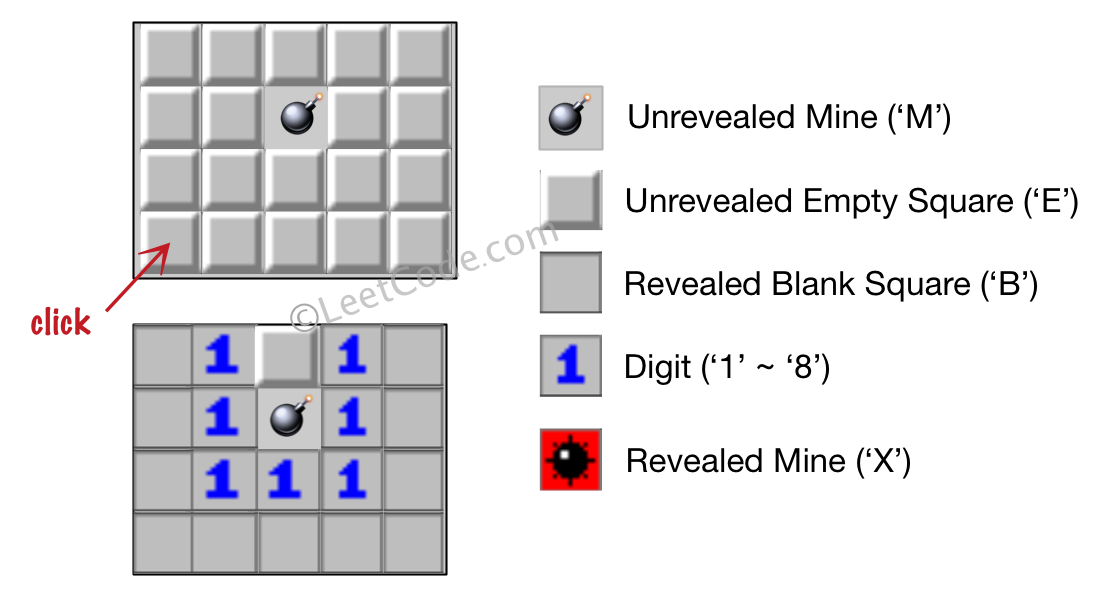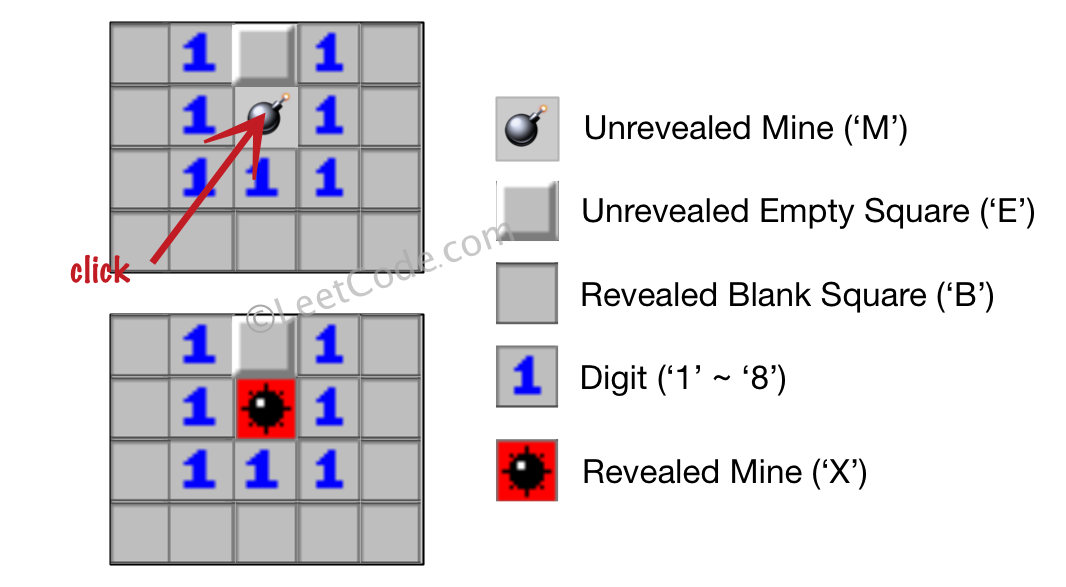## Algorithm

Problem Name: 529. Minesweeper

Let's play the minesweeper game (Wikipedia, online game)!

You are given an `m x n` char matrix `board` representing the game board where:

• `'M'` represents an unrevealed mine,
• `'E'` represents an unrevealed empty square,
• `'B'` represents a revealed blank square that has no adjacent mines (i.e., above, below, left, right, and all 4 diagonals),
• digit (`'1'` to `'8'`) represents how many mines are adjacent to this revealed square, and
• `'X'` represents a revealed mine.

You are also given an integer array `click` where `click = [clickr, clickc]` represents the next click position among all the unrevealed squares (`'M'` or `'E'`).

Return the board after revealing this position according to the following rules:

1. If a mine `'M'` is revealed, then the game is over. You should change it to `'X'`.
2. If an empty square `'E'` with no adjacent mines is revealed, then change it to a revealed blank `'B'` and all of its adjacent unrevealed squares should be revealed recursively.
3. If an empty square `'E'` with at least one adjacent mine is revealed, then change it to a digit (`'1'` to `'8'`) representing the number of adjacent mines.
4. Return the board when no more squares will be revealed.

Example 1:```Input: board = [["E","E","E","E","E"],["E","E","M","E","E"],["E","E","E","E","E"],["E","E","E","E","E"]], click = [3,0]
Output: [["B","1","E","1","B"],["B","1","M","1","B"],["B","1","1","1","B"],["B","B","B","B","B"]]
```

Example 2:```Input: board = [["B","1","E","1","B"],["B","1","M","1","B"],["B","1","1","1","B"],["B","B","B","B","B"]], click = [1,2]
Output: [["B","1","E","1","B"],["B","1","X","1","B"],["B","1","1","1","B"],["B","B","B","B","B"]]
```

Constraints:

• `m == board.length`
• `n == board[i].length`
• `1 <= m, n <= 50`
• `board[i][j]` is either `'M'`, `'E'`, `'B'`, or a digit from `'1'` to `'8'`.
• `click.length == 2`
• `0 <= clickr < m`
• `0 <= clickc < n`
• `board[clickr][clickc]` is either `'M'` or `'E'`.

## Code Examples

### #1 Code Example with Java Programming

```Code - Java Programming```

``````
class Solution {
int[][] dirs = {{1, 0}, {0, 1}, {-1, 0}, {0, -1}, {1, -1}, {-1, 1}, {1, 1}, {-1, -1}};
public char[][] updateBoard(char[][] board, int[] click) {
dfs(board, click, click);
return board;
}

private void dfs(char[][] board, int x, int y) {
if (!isCoordinateValid(x, y, board)) {
return;
}

if (board[x][y] == 'M') {
board[x][y] = 'X';
}

if (board[x][y] == 'E') {
int mineCount = getAdjacentMineCount(board, x, y);
if (mineCount == 0) {
board[x][y] = 'B';
for (int[] dir : dirs) {
dfs(board, x + dir, y + dir);
}
}
else {
board[x][y] = (char) (mineCount + '0');
}
}
}

private boolean isCoordinateValid(int x, int y, char[][] board) {
return !(x < 0 || x >= board.length || y < 0 || y >= board.length);
}

private int getAdjacentMineCount(char[][] board, int x, int y) {
int count = 0;
for (int[] dir : dirs) {
if (isCoordinateValid(x + dir, y + dir, board) && board[x + dir][y + dir] == 'M') {
count++;
}
}

return count;
}
}
``````
Copy The Code &

Input

cmd
board = [["E","E","E","E","E"],["E","E","M","E","E"],["E","E","E","E","E"],["E","E","E","E","E"]], click = [3,0]

Output

cmd
[["B","1","E","1","B"],["B","1","M","1","B"],["B","1","1","1","B"],["B","B","B","B","B"]]

### #2 Code Example with Javascript Programming

```Code - Javascript Programming```

``````
const updateBoard = function(board, click) {
const visited = new Set();
const [clickRow, clickCol] = click;
if (board[clickRow][clickCol] === "M") {
board[clickRow][clickCol] = "X";
return board;
}
const directions = [
[-1, 0], // top
[1, 0], // down
[0, -1], // left
[0, 1], // right
[-1, -1], // top left
[1, 1], // bottom right
[-1, 1], // top right
[1, -1] // bottom left
];

function dfs(row, col) {
if (board[row][col] === "M") return;
let numBombs = 0;
for (let dir of directions) {
if (
board[row + dir] === undefined ||
board[row + dir][col + dir] === undefined
)
continue;
if (board[row + dir][col + dir] === "M") {
numBombs += 1;
}
}
if (numBombs) {
board[row][col] = `\${numBombs}`;
return;
}
board[row][col] = "B";
for (let dir of directions) {
if (
board[row + dir] === undefined ||
board[row + dir][col + dir] === undefined ||
board[row + dir][col + dir] !== "E"
)
continue;
if (!visited.has(`\${row + dir},\${col + dir}`)) {
dfs(row + dir, col + dir);
}
}
}
dfs(clickRow, clickCol);
return board;
};
``````
Copy The Code &

Input

cmd
board = [["E","E","E","E","E"],["E","E","M","E","E"],["E","E","E","E","E"],["E","E","E","E","E"]], click = [3,0]

Output

cmd
[["B","1","E","1","B"],["B","1","M","1","B"],["B","1","1","1","B"],["B","B","B","B","B"]]

### #3 Code Example with Python Programming

```Code - Python Programming```

``````
class Solution(object):
def updateBoard(self, board, click):
def explore(i, j):
if board[i][j] == "M": board[i][j] = "X"
else:
points = ((i - 1, j), (i + 1, j), (i, j - 1), (i, j + 1), (i - 1, j - 1), (i - 1, j + 1), (i + 1, j + 1), (i + 1, j - 1))
for p in points:
if 0 <= p < m and 0 <= p < n:
if board[p][p] == "M": cnt += 1
elif p not in visited: adj += p,
if cnt == 0:
board[i][j] = "B"
for p in adj: explore(p, p)
else: board[i][j] = str(cnt)
m, n, visited = len(board), len(board and board), set()
explore(click, click)
return board
``````
Copy The Code &

Input

cmd
board = [["B","1","E","1","B"],["B","1","M","1","B"],["B","1","1","1","B"],["B","B","B","B","B"]], click = [1,2]

Output

cmd
[["B","1","E","1","B"],["B","1","X","1","B"],["B","1","1","1","B"],["B","B","B","B","B"]]

### #4 Code Example with C# Programming

```Code - C# Programming```

``````
using System.Collections.Generic;

namespace LeetCode
{
public class _0529_Minesweeper
{
private static readonly (int dr, int dc)[] directions = { (0, 1), (0, -1), (1, 0), (-1, 0), (1, 1), (1, -1), (-1, 1), (-1, -1) };

public char[][] UpdateBoard(char[][] board, int[] click)
{
if (board[click][click] == 'M')
{
board[click][click] = 'X';
return board;
}

int N = board.Length;
int M = board.Length;

var visisted = new HashSet<(int r, int c)>();
var queue = new Queue<(int r, int c)>();
queue.Enqueue((click, click));

var adjList = new List<(int r, int c)>();
while (queue.Count > 0)
{
(int r, int c) = queue.Dequeue();
if (visisted.Contains((r, c))) continue;

board[r][c] = 'B';

var count = 0;
foreach (var dir in directions)
{
var newR = r + dir.dr;
var newC = c + dir.dc;

if (newR < 0 || newR >= N || newC < 0 || newC >= M) continue;

if (board[newR][newC] == 'E')
if (board[newR][newC] == 'M')
count++;
}

if (count == 0)
foreach ((int newR, int newC) in adjList)
queue.Enqueue((newR, newC));
else
board[r][c] = (char)('0' + count);
}

return board;
}
}
}
``````
Copy The Code &

Input

cmd
board = [["B","1","E","1","B"],["B","1","M","1","B"],["B","1","1","1","B"],["B","B","B","B","B"]], click = [1,2]

Output

cmd
[["B","1","E","1","B"],["B","1","X","1","B"],["B","1","1","1","B"],["B","B","B","B","B"]]